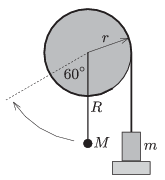Mathematical and Physical Journal
for High Schools
Issued by the MATFUND Foundation
 Already signed up? New to KöMaL?

#Problem P. 4655. (September 2014)

P. 4655. A piece of thread is wound around the rim of a very light disc if radius $\displaystyle r=0.1$ m. An object of mass $\displaystyle m=100$ g is attached to the free end of the thread. The disc has a horizontal axle at its centre and also there is a rod of negligible mass fixed to it. There is a small object of mass $\displaystyle M$ attached to the end of the rod at a distance of $\displaystyle R=0.2$ m from the centre of the disc.

Initially the system is at rest in the position shown in the figure. Suddenly the support of the object of mass $\displaystyle m$ is taken away, and the small object of mass $\displaystyle M$ just reaches the position indicated by the broken line, enclosing a $\displaystyle 60^\circ$ angle with the vertical. After some lightly damped swings the rod reaches its equilibrium position, at which the angle between the rod and the vertical is $\displaystyle \varphi$.$\displaystyle a)$ What is the mass $\displaystyle M$ of the object at the end of the rod?

$\displaystyle b)$ What is the measure of the angle $\displaystyle \varphi$?

$\displaystyle c)$ If the system is displaced a bit from its equilibrium position, what is the period of the oscillation?

(5 pont)

Deadline expired on October 10, 2014.

### Statistics:

 58 students sent a solution. 5 points: Asztalos Bogdán, Balogh Menyhért, Berta Dénes, Blum Balázs, Forrai Botond, Kasza Bence, Kovács Péter Tamás, Molnár 128 Szilárd, Olosz Balázs, Öreg Botond, Sáfrán Péter, Sal Kristóf, Südi István, Tompa Tamás Lajos. 4 points: 38 students. 3 points: 2 students. 2 points: 1 student. 1 point: 2 students. 0 point: 1 student.

Problems in Physics of KöMaL, September 2014